Banking is the business of receiving, safeguarding and lending of money. In this Chapter, students are introduced to Recurring Deposit Account and computation of its maturity value. Those students wanting more clarity to solve the problems of any chapter in the textbook can make use of Selina Solutions. All these solutions are prepared by subject experts at BYJU’S according to the latest examination patterns.

In Chapter 2 of Selina Solutions Concise Maths Class 10, there are two exercises. Problems based on computing the maturity value of a RD account is the prime focus in these exercises. For strong preparations, students can also access the Selina Solutions Concise Maths Class 10 Chapter 2 Banking (Recurring Deposit Accounts)PDF from the links provided below.

## Selina Solutions Concise Maths Class 10 Chapter 2 Banking (Recurring Deposit Accounts) Download PDF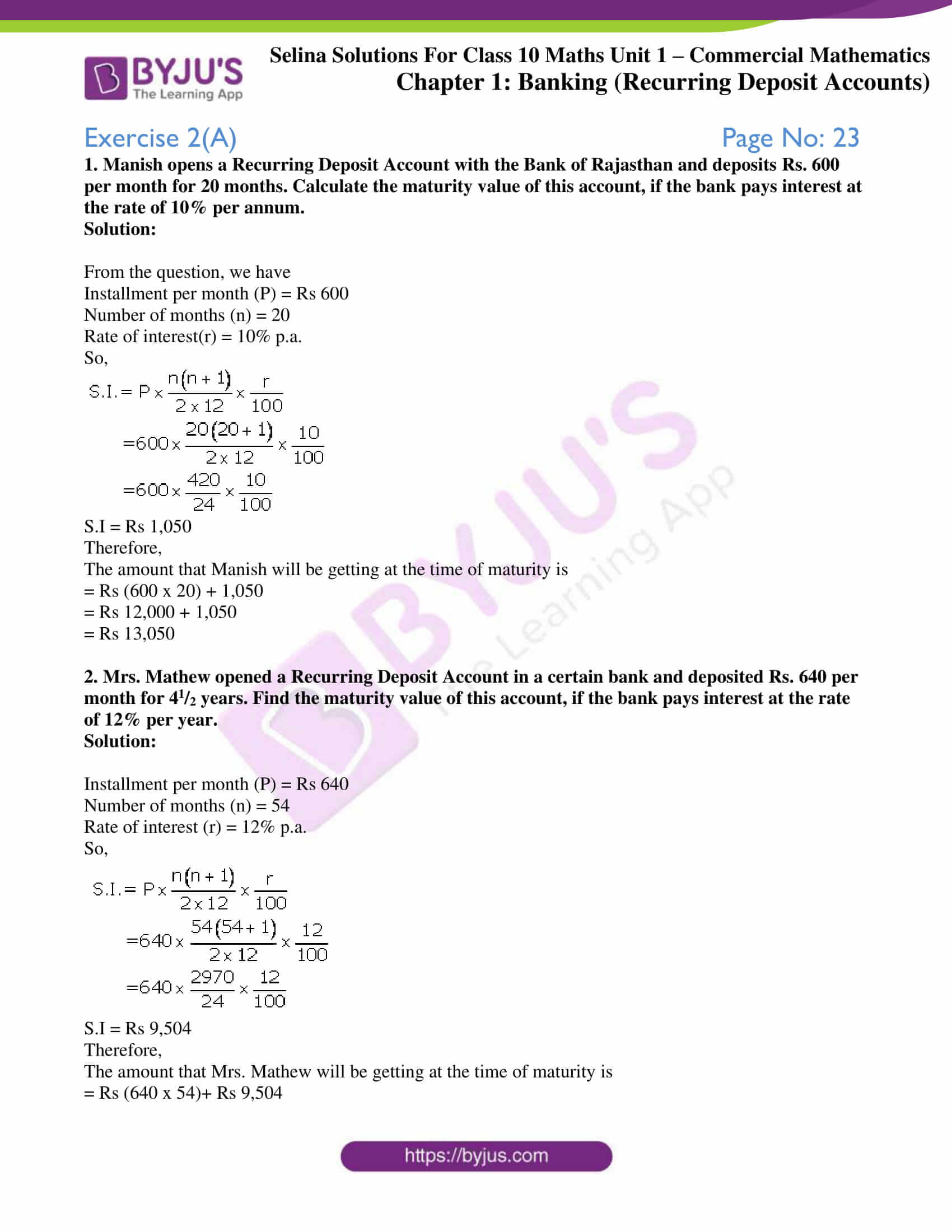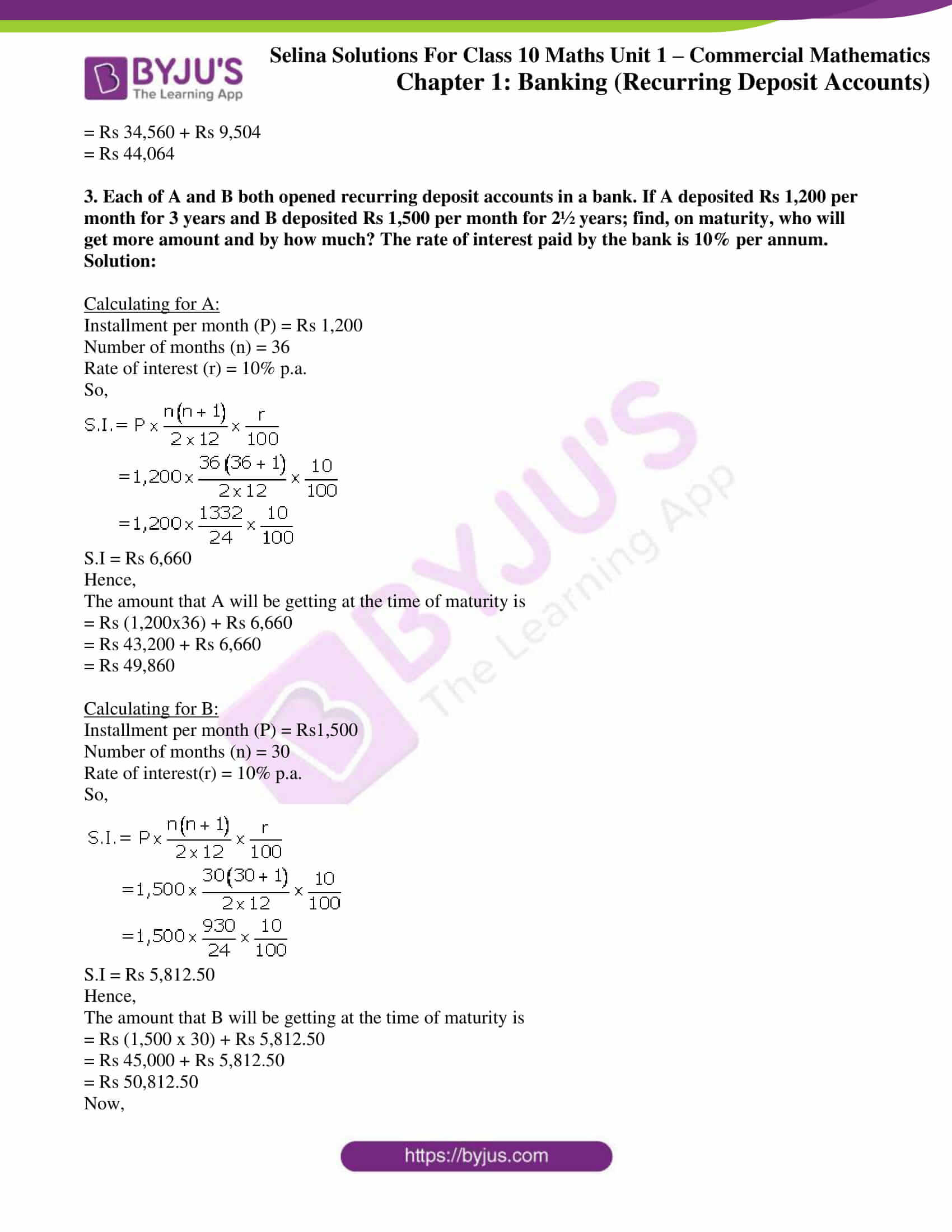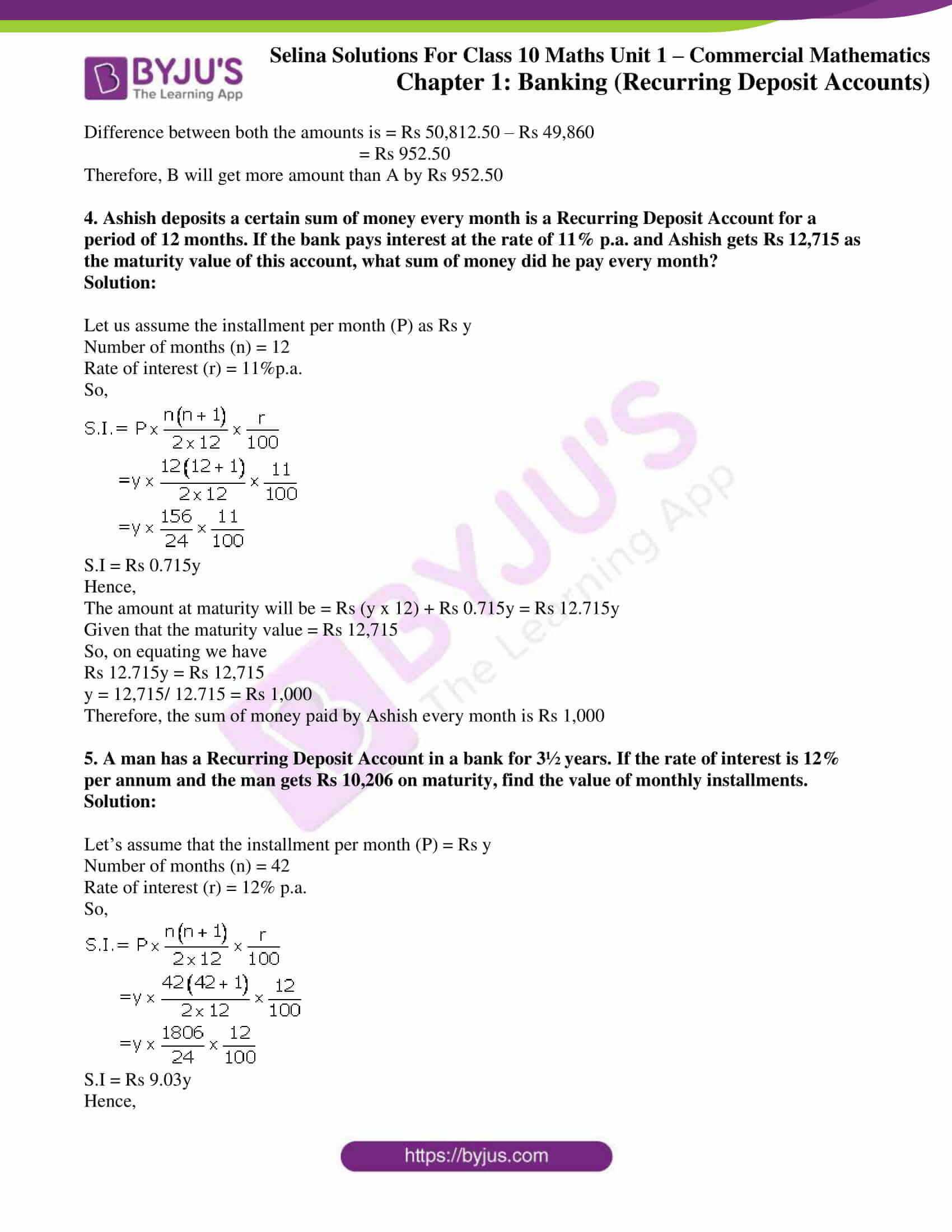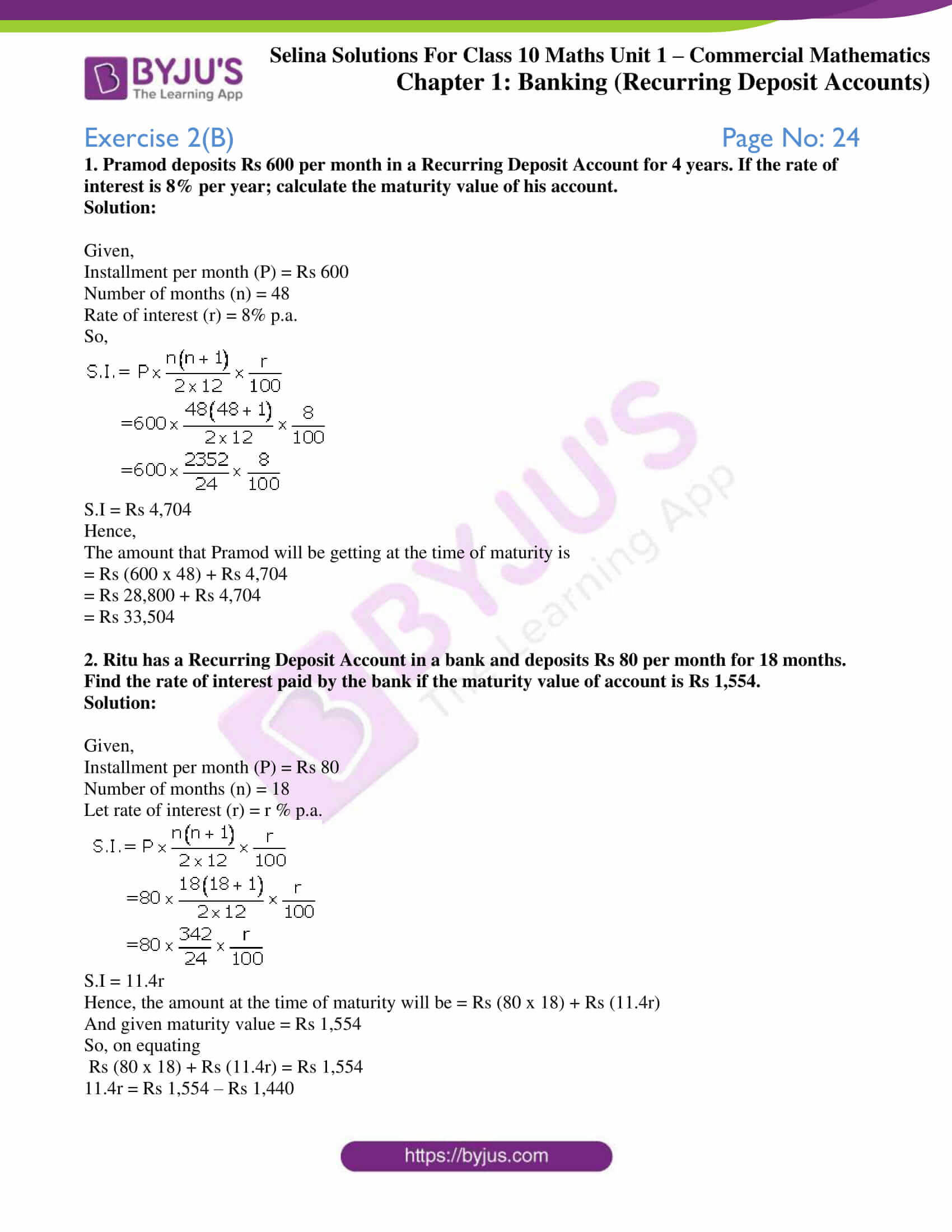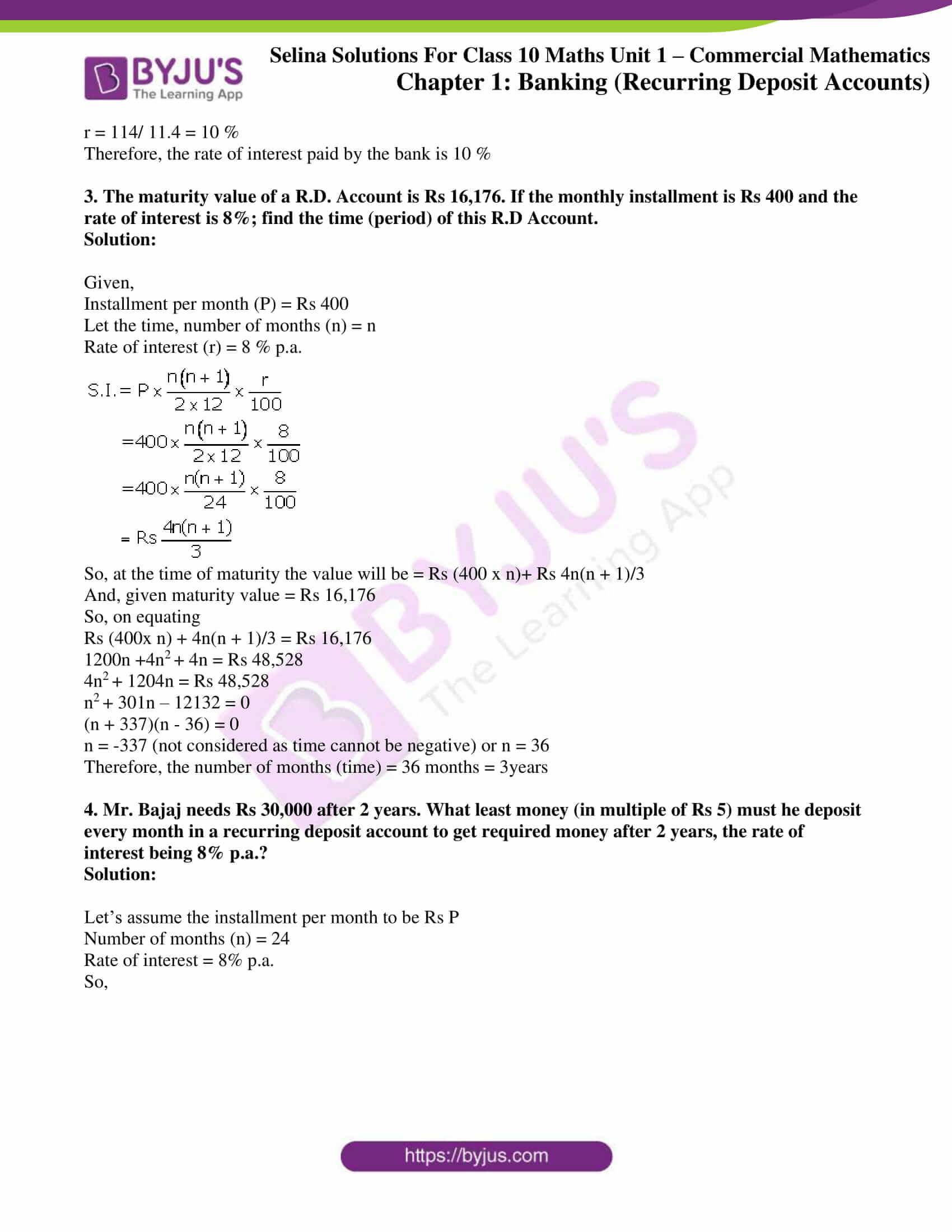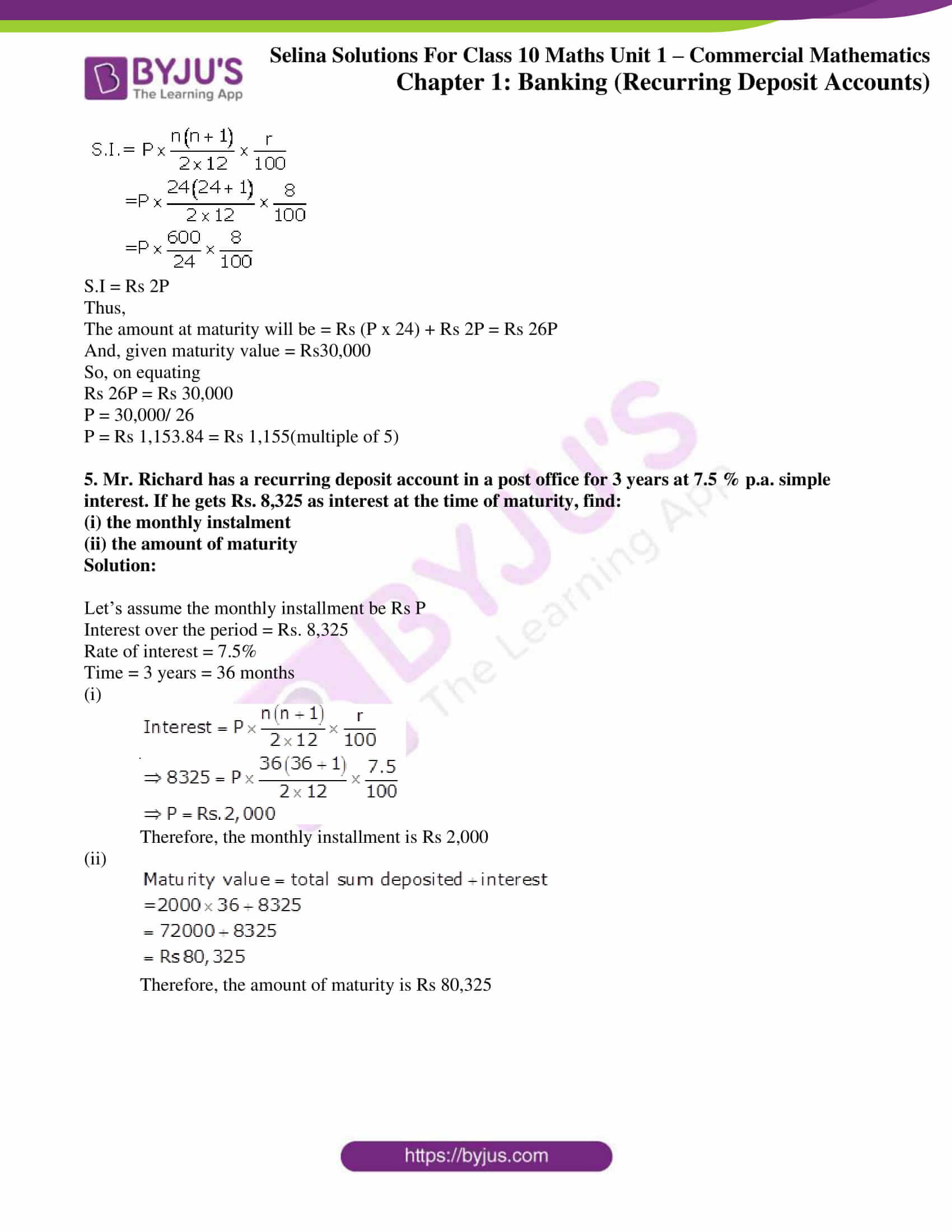## Access Selina Solutions Concise Maths Class 10 Chapter 2 Banking (Recurring Deposit Accounts)

### Exercise 2(A) Page No: 23

1. Manish opens a Recurring Deposit Account with the Bank of Rajasthan and deposits Rs. 600 per month for 20 months. Calculate the maturity value of this account, if the bank pays interest at the rate of 10% per annum.

Solution:

From the question, we have

Installment per month (P) = Rs 600

Number of months (n) = 20

Rate of interest(r) = 10% p.a.

So,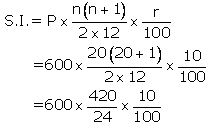S.I = Rs 1,050

Therefore,

The amount that Manish will be getting at the time of maturity is

= Rs (600 x 20) + 1,050

= Rs 12,000 + 1,050

= Rs 13,050

2. Mrs. Mathew opened a Recurring Deposit Account in a certain bank and deposited Rs. 640 per month for 41/2 years. Find the maturity value of this account, if the bank pays interest at the rate of 12% per year.

Solution:

Installment per month (P) = Rs 640

Number of months (n) = 54

Rate of interest (r) = 12% p.a.

So,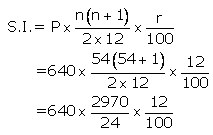S.I = Rs 9,504

Therefore,

The amount that Mrs. Mathew will be getting at the time of maturity is

= Rs (640 x 54)+ Rs 9,504

= Rs 34,560 + Rs 9,504

= Rs 44,064

3. Each of A and B both opened recurring deposit accounts in a bank. If A deposited Rs 1,200 per month for 3 years and B deposited Rs 1,500 per month for 2½ years; find, on maturity, who will get more amount and by how much? The rate of interest paid by the bank is 10% per annum.

Solution:

Calculating for A:

Installment per month (P) = Rs 1,200

Number of months (n) = 36

Rate of interest (r) = 10% p.a.

So,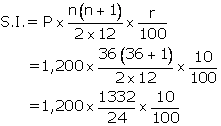S.I = Rs 6,660

Hence,

The amount that A will be getting at the time of maturity is

= Rs (1,200×36) + Rs 6,660

= Rs 43,200 + Rs 6,660

= Rs 49,860

Calculating for B:

Installment per month (P) = Rs1,500

Number of months (n) = 30

Rate of interest(r) = 10% p.a.

So,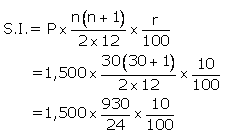S.I = Rs 5,812.50

Hence,

The amount that B will be getting at the time of maturity is

= Rs (1,500 x 30) + Rs 5,812.50

= Rs 45,000 + Rs 5,812.50

= Rs 50,812.50

Now,

Difference between both the amounts is = Rs 50,812.50 – Rs 49,860

= Rs 952.50

Therefore, B will get more amount than A by Rs 952.50

4. Ashish deposits a certain sum of money every month is a Recurring Deposit Account for a period of 12 months. If the bank pays interest at the rate of 11% p.a. and Ashish gets Rs 12,715 as the maturity value of this account, what sum of money did he pay every month?

Solution:

Let us assume the installment per month (P) as Rs y

Number of months (n) = 12

Rate of interest (r) = 11%p.a.

So,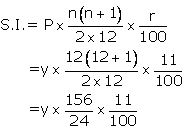S.I = Rs 0.715y

Hence,

The amount at maturity will be = Rs (y x 12) + Rs 0.715y = Rs 12.715y

Given that the maturity value = Rs 12,715

So, on equating we have

Rs 12.715y = Rs 12,715

y = 12,715/ 12.715 = Rs 1,000

Therefore, the sum of money paid by Ashish every month is Rs 1,000

5. A man has a Recurring Deposit Account in a bank for 3½ years. If the rate of interest is 12% per annum and the man gets Rs 10,206 on maturity, find the value of monthly installments.

Solution:

Let’s assume that the installment per month (P) = Rs y

Number of months (n) = 42

Rate of interest (r) = 12% p.a.

So,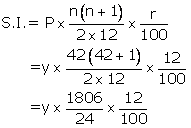S.I = Rs 9.03y

Hence,

The amount at maturity will be = Rs (y x 42) + Rs 9.03y = Rs 51.03y

But given maturity value = Rs10,206

So, on equating we have

Rs 51.03y = Rs 10206

y = 10206/ 51.03 = Rs 200

Therefore, the value of monthly installment is Rs 200

### Exercise 2(B) Page No: 24

1. Pramod deposits Rs 600 per month in a Recurring Deposit Account for 4 years. If the rate of interest is 8% per year; calculate the maturity value of his account.

Solution:

Given,

Installment per month (P) = Rs 600

Number of months (n) = 48

Rate of interest (r) = 8% p.a.

So,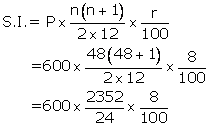S.I = Rs 4,704

Hence,

The amount that Pramod will be getting at the time of maturity is

= Rs (600 x 48) + Rs 4,704

= Rs 28,800 + Rs 4,704

= Rs 33,504

2. Ritu has a Recurring Deposit Account in a bank and deposits Rs 80 per month for 18 months. Find the rate of interest paid by the bank if the maturity value of account is Rs 1,554.

Solution:

Given,

Installment per month (P) = Rs 80

Number of months (n) = 18

Let rate of interest (r) = r % p.a.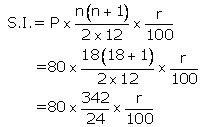S.I = 11.4r

Hence, the amount at the time of maturity will be = Rs (80 x 18) + Rs (11.4r)

And given maturity value = Rs 1,554

So, on equating

Rs (80 x 18) + Rs (11.4r) = Rs 1,554

11.4r = Rs 1,554 – Rs 1,440

r = 114/ 11.4 = 10 %

Therefore, the rate of interest paid by the bank is 10 %

3. The maturity value of a R.D. Account is Rs 16,176. If the monthly installment is Rs 400 and the rate of interest is 8%; find the time (period) of this R.D Account.

Solution:

Given,

Installment per month (P) = Rs 400

Let the time, number of months (n) = n

Rate of interest (r) = 8 % p.a.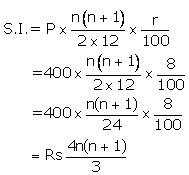So, at the time of maturity the value will be = Rs (400 x n)+ Rs 4n(n + 1)/3

And, given maturity value = Rs 16,176

So, on equating

Rs (400x n) + 4n(n + 1)/3 = Rs 16,176

1200n +4n2 + 4n = Rs 48,528

4n2 + 1204n = Rs 48,528

n2 + 301n – 12132 = 0

(n + 337)(n – 36) = 0

n = -337 (not considered as time cannot be negative) or n = 36

Therefore, the number of months (time) = 36 months = 3years

4. Mr. Bajaj needs Rs 30,000 after 2 years. What least money (in multiple of Rs 5) must he deposit every month in a recurring deposit account to get required money after 2 years, the rate of interest being 8% p.a.?

Solution:

Let’s assume the installment per month to be Rs P

Number of months (n) = 24

Rate of interest = 8% p.a.

So,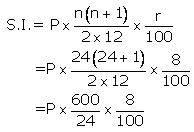S.I = Rs 2P

Thus,

The amount at maturity will be = Rs (P x 24) + Rs 2P = Rs 26P

And, given maturity value = Rs30,000

So, on equating

Rs 26P = Rs 30,000

P = 30,000/ 26

P = Rs 1,153.84 = Rs 1,155(multiple of 5)

5. Mr. Richard has a recurring deposit account in a post office for 3 years at 7.5 % p.a. simple interest. If he gets Rs. 8,325 as interest at the time of maturity, find:

(i) the monthly instalment

(ii) the amount of maturity

Solution:

Let’s assume the monthly installment be Rs P

Interest over the period = Rs. 8,325

Rate of interest = 7.5%

Time = 3 years = 36 months

(i)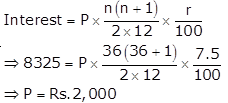Therefore, the monthly installment is Rs 2,000

(ii)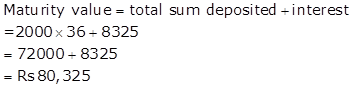Therefore, the amount of maturity is Rs 80,325

### Exercises of Selina Solutions Concise Maths Class 10 Chapter 2 Banking (Recurring Deposit Accounts)

Exercise 2(A) Solutions

Exercise 2(B) Solutions